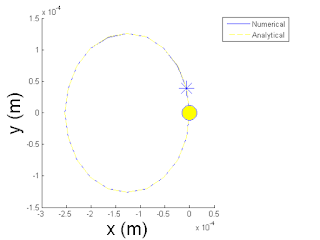## Friday, July 8, 2016

### Space Physics: Cyclotron Motion

I'll discuss a basic topic in Space Physics today that is certainly not a basic idea for the average person outside of science to understand.  However! that should not matter nor should it intimidate you!  Any person (such as myself) that attempts to teach ANY scientific concept should be capable of explaining their science in such a way that even children with little scientific background can understand the concept.(Credit: spaceweather.com)
The basic topic is the behavior of a proton as it approaches the Earth's magnetic field.  Remember, an atom--those boxes you see on a periodic table--can consist of a positively charged proton and a negatively charged electron.  Because I am discussing a topic in the field of space physics, I will also remind you that the Earth's magnetic field is always being hit by protons and electrons from the Sun.

Today, I will describe one of the ways a proton behaves when encountering an unchanging magnetic field line.

When a proton approaches a magnetic field line, the proton spirals around a magnetic field line and continues in the direction of that line.  This is known as cyclotron motion.Top View
The video illustrates a simulation in which a magnetic field line is aligned with the z-axis and is pointing upward in the +z direction (also denoted as the $$+\hat{\textbf{e}}_z$$ direction).  This is why the proton travels in the +z direction over time while moving in a circle.  Mathematically, the +z direction of the particle's motion is shown via the $$v_{\|}t\hat{\textbf{e}}_z$$ term at the end of my cyclotron motion derivation.

The image to the right provides a top view of the simulation.  This perspective allowed me to verify if the proton truly has a circular trajectory in 2D space.  It is easy to see (thanks to the numbers on the axes) that the proton traveled 0.23 meters in the +y direction as well as the -y direction.  The trajectory looks visually like an ellipse (ugghhhh, thanks MATLAB...) but after looking at the numbers, I found that the proton truly did travel 0.23 meters from the center of the circle in all directions.  The radius of this circular cyclotron motion is known as the Larmor orbital radius:
$r_L = \Big| \frac{v_{\perp}}{\Omega} \Big| = \Big| \frac{mv_{\perp}}{qB} \Big|$
where $$\Omega$$ is the frequency of the orbit, m is the mass of the particle (in this case a proton), $$v_{\perp}$$ is the component of velocity of the particle that goes in a direction that is perpendicular to the magnetic field B, and q is the charge of that particle (in this case +1.602$$\times$$10$$^{-19}$$ Coulombs).

Now, let's see how the electron behaves in the presence of a magnetic field line.  Notice anything different?Top View
Hmmmm... Interesante.  At first glance, it seems to have done the same thing as the proton.  One difference seen is that the electron's cyclotron motion is counterclockwise while the proton's motion is clockwise.  Another distinction is noticed when looking at the simulation from the top view.  The Larmor radius is now 1.26$$\times$$ 10$$^{-4}$$ meters.

Mathematically speaking, the reason why the electron's Larmor radius is shorter than the proton's Larmor radius is because the mass of an electron is about 1800 times less than the mass of a proton, and because the $$r_L$$ is directly proportional to the m, the super tiny electron mass makes its Larmor radius super tiny as well.

The difference in clockwise vs counterclockwise motion between proton and electron is due to the Lorentz force:
$\vec{F} = q(\vec{E} + \vec{v} \times \vec{B} )$
where $$\vec{E}$$ is the electric field.  Basically, this equation means that whenever a charged particle is in the presence of an electric or magnetic field, a force will be applied to that particle which will cause it to either to accelerate or change directions.  The arrows on top of the variables indicate that they are vectors, which means that the electric field strength, the particle's velocity, and the magnetic field strength are all pointed in some direction.  In my simulation, I have not included an electric field.  Therefore, in this case, $$\vec{E}=0$$ and $$\vec{F} = q ( \vec{v} \times \vec{B} )$$.  Between the proton and electron simulations I did not change the velocity nor the magnetic field.  The only thing that was different is the charge q.  Since protons and electrons have opposite charge, the magnetic field's force $$\vec{F}$$ was pushing them in opposite directions!  Before clicking on my previous videos, you could have predicted if the motion was going to be clockwise or counterclockwise as long as you would have used the right hand rule for the cross product $$\vec{v} \times \vec{B}$$.

Later, I will discuss other behaviors that particles from the Sun exhibit when they encounter the Earth's magnetic field.

Deriving Position from Velocity of Pure Cyclotron Motion
\begin{equation} \textbf{v}(t) = v_{\perp}sin(\Omega_c t)\hat{\textbf{e}}_x + v_{\perp}cos(\Omega_c t)\hat{\textbf{e}}_y + v_{\|}\hat{\textbf{e}}_z
\end{equation}
$\int_{t=0}^{t} \textbf{v}(t) dt = \int_{t=0}^{t} \big( v_{\perp}sin(\Omega_c t)\hat{\textbf{e}}_x + v_{\perp}cos(\Omega_c t)\hat{\textbf{e}}_y + v_{\|}\hat{\textbf{e}}_z \big)dt$
$\textbf{r}(t)-\textbf{r}(0) = -\frac{v_{\perp}}{\Omega_c}[cos(\Omega_c t) - 1]\hat{\textbf{e}}_x + \frac{v_{\perp}}{\Omega_c}[sin(\Omega_c t)- 0]\hat{\textbf{e}}_y + v_{\|}[t - 0]\hat{\textbf{e}}_z$
\begin{equation}\label{Eq2: Analy path} \textbf{r}(t)-\textbf{r}(0) = \frac{v_{\perp}}{\Omega_c}[1 - cos(\Omega_c t)]\hat{\textbf{e}}_x + \frac{v_{\perp}}{\Omega_c}sin(\Omega_c t)\hat{\textbf{e}}_y + v_{\|}t\hat{\textbf{e}}_z
\end{equation}